PATTERNS OF OCEAN CIRCULATION OVER THE CONTINENTS DURING NOAH'S FLOOD
Click on any of the figures below to get a larger image.
 Figure 1. Computational mesh constructed from icosahedron. Mesh has 40962 nodes. Nodes are located at centers of hexagonal cells. Node spacing is about 125km. Figure 2. Snapshots from solutin of the shallow water equations for a bottom topography corresponding to a Pangean-like continent initially flooded to a depth of 500 meters. Initial water depth contours are shown in (a) with the minimum contour equal to 500 m and the maximum contour equal to 3980 m. The initial velocities are everywhere zero. Frames (b) - (g) show the solution at 10-day intervals.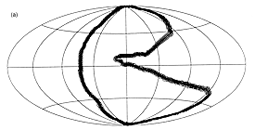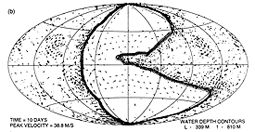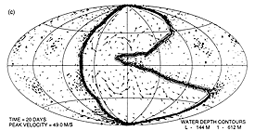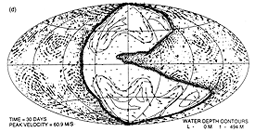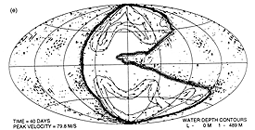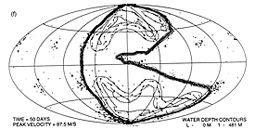Figure 3. Snapshots at 80 days (a) and 90 days (b) from calculation identical to that of Fig. 2 except initial water depth over the continent is 1000 m and over oceanic regions is 3680 m. Solution displays strong time vatiation.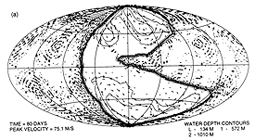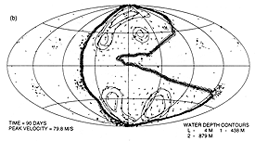Figure 4. Snapshots at 70 days (a) and 80 days (b) from calculation identical to that of Fig.2 except initial water depth over the continent is 1500 m and over oceanic regions is 3380 m. Solution displays moderate time vatiation.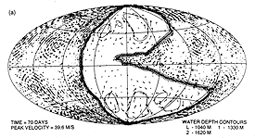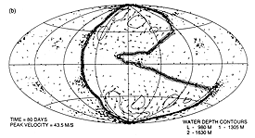Figure 5. Snapshot at a time of 80 days from a solution of the shallow water equations with a bottom topography consisting of a circular continent 5560 km in diameter centered at 45 degrees latitude in each hemisphere and initially flooded to a depth of 1000 meters. Initial water depth in the oceanic areas is 3000 m. Figure 6. Snapshot at 40 days for a case identical to that of Fig. 5 except the continent diameter is 3330 km and the initial flooding depth is 250 m.